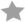# The Hunter

## Acordes

##### Danzig (1988)

Más Versiones```THE HUNTER
==========
Note: (G) is a bent note on the third fret, sixth string.

A
A  C D  A  D C (2x) w/solo
D  F E  D  F C      w/solo
A  C D  A  D C      w/solo
E  D  C             w/solo
E  (G)
A  C D  A  D C (2x)
D  F E  D  F C
A  C D  A  D C
E  D  C
E  (G)
A  C D  A  D C (2x) w/solo
D  F E  D  F C      w/solo
A  C D  A  D C      w/solo
E  D  C             w/solo
E  (G)
A  C D  A  D C (2x)
D  F E  D  F C
A  C D  A  D C
E  D  C
E  (G)
A  C D  A  D C (2x) w/solo
D  F E  D  F C      w/solo
A  C D  A  D C      w/solo
E  D  C             w/solo
E```